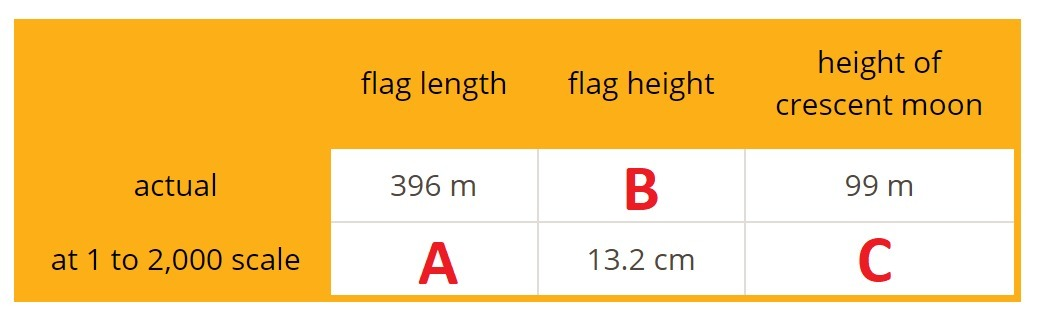PSA9CXH - Student-Facing Tasks---7.1 Lesson 12: Units in Scale Drawings (7.G.A.1)
Part A)

There are 2.54 cm in an inch, 12 inches in a foot, and 5,280 feet in a mile. Which expression gives the number of centimeters in a mile?

A.B.C.D.E.Select one:
Part B)

Part A)

Your teacher will give you some cards with a scale on each card.

Sort the cards into sets of equivalent scales. Be prepared to explain how you know that the scales in each set are equivalent. Each set should have at least two cards.

Trade places with another group and check each other’s work. If you disagree about how the scales should be sorted, work to reach an agreement.

Part B)

Next, record one of the sets with three equivalent scales and explain why they are equivalent.

Part A)

As of 2016, Tunisia holds the world record for the largest version of a national flag. It was almost as long as four soccer fields. The flag has a circle in the center, a crescent moon inside the circle, and a star inside the crescent moon.

Complete the table.What value goes in cell A?

Type your answer below as a number (example: 5, 3.1, 4 1/2, or 3/2):
Part B)

What value goes in cell B?

Type your answer below as a number (example: 5, 3.1, 4 1/2, or 3/2):
Part C)

What value goes in cell C?

Type your answer below as a number (example: 5, 3.1, 4 1/2, or 3/2):
Part D)

Part E)

Complete each scale with the value that makes it equivalent to the scale of 1 to 2,000.

1 cm to ____ cm

Type your answer below as a number (example: 5, 3.1, 4 1/2, or 3/2):
Part F)

Part G)

1 cm to ____ m

Type your answer below as a number (example: 5, 3.1, 4 1/2, or 3/2):
Part H)

Part I)

1 cm to ____ km

Type your answer below as a number (example: 5, 3.1, 4 1/2, or 3/2):
Part J)

Part K)

2 m to _____ m

Type your answer below as a number (example: 5, 3.1, 4 1/2, or 3/2):
Part L)

Part M)

5 cm to ____ m

Type your answer below as a number (example: 5, 3.1, 4 1/2, or 3/2):
Part N)

Part O)

____ cm to 1,000 m

Type your answer below as a number (example: 5, 3.1, 4 1/2, or 3/2):
Part P)

Part Q)

____ mm to 20 m

Type your answer below as a number (example: 5, 3.1, 4 1/2, or 3/2):
Part R)

Part S)

What is the area of the large flag?

Type your answer below as a number (example: 5, 3.1, 4 1/2, or 3/2):
Part T)

What is the area of the smaller flag?

Type your answer below as a number (example: 5, 3.1, 4 1/2, or 3/2):
Part U)

The area of the large flag is how many times the area of the smaller flag?

Type your answer below as a number (example: 5, 3.1, 4 1/2, or 3/2):
Part A)

Your teacher will give you a floor plan of a recreation center.

What is the scale of the floor plan if the actual side length of the square pool is 15 m? Express your answer as a scale with units.

Part B)

3:4

with no spaces.

Part C)

Find the actual area of the large rectangular pool.

Type your answer below as a number (example: 5, 3.1, 4 1/2, or 3/2):
Part D)

Part E)

The kidney-shaped pool has an area of 3.2 cm2 on the drawing. What is its actual area?

Type your answer below as a number (example: 5, 3.1, 4 1/2, or 3/2):
Part F)

Part A)

Lin and her brother each created a scale drawing of their backyard, but at different scales. Lin used a scale of 1 inch to 1 foot. Her brother used a scale of 1 inch to 1 yard.

Express Lin's scale for her drawing without units.

3:4

with no spaces.

Part B)

Express Lin's brother's scale for his drawing without units.

3:4

with no spaces.

Part C)

Whose drawing is larger?

Select one:
Part D)

How many times as large is it?

Type your answer below as a number (example: 5, 3.1, 4 1/2, or 3/2):
Part E)